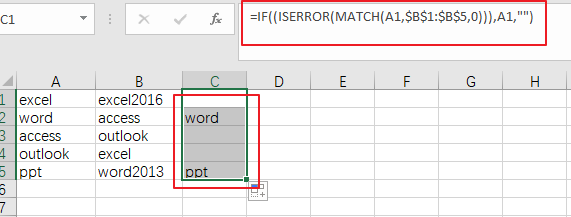# How to Compare Two Columns for Differences in Excel

This post will guide you how to compare two columns and return differences in Excel. How do I compare two columns to find differences in Excel 2013/2016.

## Compare Two Columns for Differences

Assuming that you have a list of data in range A1:B5, and you want to compare Column A and Column B and return the differences from these two columns. You can use an formula based on the IF function, the ISERROR function and the MATCH function to compare those two columns and find the differences.  like this:

if you want to get the differences between column A and Column B, just use the following formula:

=IF((ISERROR(MATCH(A1,\$B\$1:\$B\$5,0))),A1,””)

type this formula into a blank cell and press Enter key on your keyboard, and then drag the AutoFill Handle down to other cells.  You would see the differences.### Related Functions

• Excel IF function
The Excel IF function perform a logical test to return one value if the condition is TRUE and return another value if the condition is FALSE. The IF function is a build-in function in Microsoft Excel and it is categorized as a Logical Function.The syntax of the IF function is as below:= IF (condition, [true_value], [false_value])….
• Excel IFERROR function
The Excel IFERROR function returns an alternate value you specify if a formula results in an error, or returns the result of the formula.The syntax of the IFERROR function is as below:= IFERROR (value, value_if_error)….
• Excel MATCH function
The Excel MATCH function search a value in an array and returns the position of that item.The syntax of the MATCH function is as below:= MATCH  (lookup_value, lookup_array, [match_type])….

Related Posts

VLOOKUP From Another Sheet Not Working

In the previous post, you should know that how to fix or remove the #N/A error when using VLOOKUP formula to lookup value from another sheet. And this post will show you reasons why your VLOOKUP formula is not working ...

Case Sensitive Lookup with SUMPRODUCT and EXACT

Today, we will show you how to use SUMPRODUCT and EXACT to perform a case sensitive exact match. In this article, we provide a simple example to calculate bonus for employees whose names are case-sensitive. If you meet similar scenarios ...

Basic Usage of INDEX & MATCH – Case Sensitive Lookup

In Excel, INDEX function and MATCH function are often used together for retrieving data from a particular position. MATCH function is one of Excel lookup & reference functions that can perform approximate match or exact match by setting different match ...

Basic Rates Calculation by VLOOKUP Based on Weight Band

Microsoft Excel provides many functions that can execute logical test, search data, return current date and something else. They are very useful in daily work. And Excel VLOOKUP function is one of Excel most frequently used functions. It belongs to ...

Basic Grade Calculation by VLOOKUP Function – Approximate Match

In Excel, except combination INDEX+MATCH, we can also apply other functions to search data, for example VLOOKUP function. Like MATCH function, VLOOKUP function is one of Excel lookup & reference functions that can perform approximate match or exact match by ...

Basic Discount Calculation with VLOOKUP Function

In Excel, except combination INDEX+MATCH, we can also apply other functions to search data, for example VLOOKUP function. Like MATCH function, VLOOKUP function is one of Excel lookup & reference functions that can perform approximate match or exact match by ...

Basic Usage of INDEX & MATCH – Exact Match

In Excel, INDEX function and MATCH function are often used together for returning value or cell reference or range reference from specified position. And MATCH function is one of Excel lookup & reference functions that can perform approximate match or ...

Basic Usage of INDEX & MATCH – Approximate Match

In Excel, INDEX function and MATCH function are often used together for returning value or cell reference or range reference from specified position. And MATCH function is one of Excel lookup & reference functions that can perform approximate match by ...

Approximate Match with Multiple Criteria by INDEX & MATCH

In Excel, INDEX function and MATCH function are often used together for returning data from specific position. And MATCH function is one of Excel lookup & reference functions that can return approximate value by setting match type. Above all, through ...

How to Count Cells Not Equal to One of Many Things in Excel

This post will guide you how to count the number of cells that are not equal to many things in a given range cells using a formula in Excel 2013/2016. You can easily to count cells not equal to a ...

Sidebar